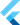# Arithmetic and Comparison Operators

on Saturday, 18th of July, 2020

Dart supports all the operators you'd expect from a modern programming language.

### Arithmetic

OperatorMeaning
+Add
-Subtract
-exprUnary minus, also known as negation (reverse the sign of the expression)
*Multiply
/Divide
~/Divide, returning an integer result
%Get the remainder of an integer division (modulo)
++varvar = var + 1 (expression value is var + 1)
var++var = var + 1 (expression value is var)
--varvar = var – 1 (expression value is var – 1)
var--var = var – 1 (expression value is var)

example use:

``````assert(2 + 3 == 5);
assert(2 - 3 == -1);
assert(2 * 3 == 6);
assert(5 / 2 == 2.5); // Result is a double
assert(5 ~/ 2 == 2); // Result is an int
assert(5 % 2 == 1); // Remainder

assert('5/2 = \${5 ~/ 2} r \${5 % 2}' == '5/2 = 2 r 1');

var a, b;

a = 0;
b = ++a; // Increment a before b gets its value.
assert(a == b); // 1 == 1

a = 0;
b = a++; // Increment a AFTER b gets its value.
assert(a != b); // 1 != 0

a = 0;
b = --a; // Decrement a before b gets its value.
assert(a == b); // -1 == -1

a = 0;
b = a--; // Decrement a AFTER b gets its value.
assert(a != b); // -1 != 0``````

### Comparison

OperatorMeaning
==Equal
!=Not equal
>Greater than
<Less than
>=Greater than or equal to
<=Less than or equal to

example usage:

``````assert(2 == 2);
assert(2 != 3);
assert(3 > 2);
assert(2 < 3);
assert(3 >= 3);
assert(2 <= 3);``````